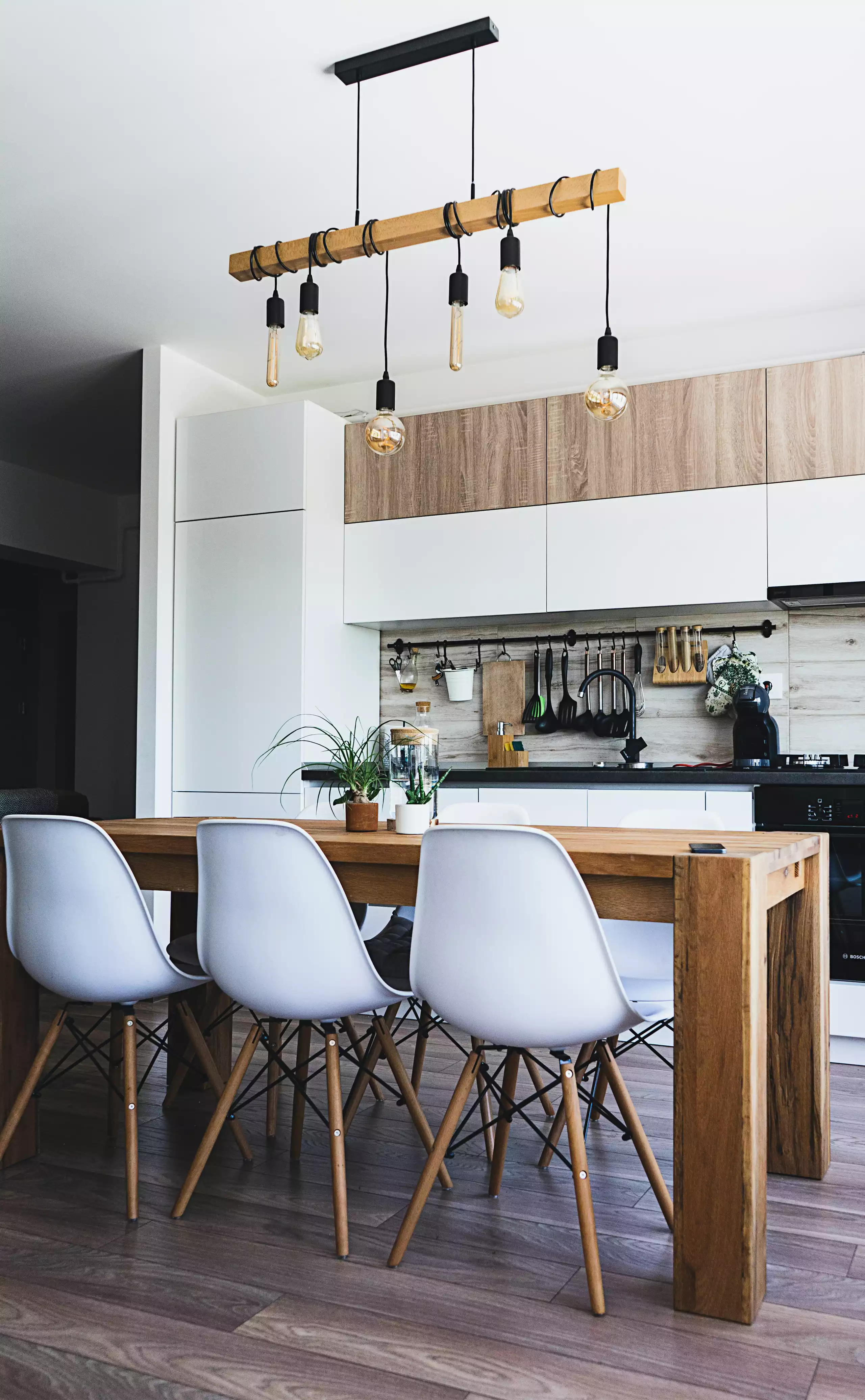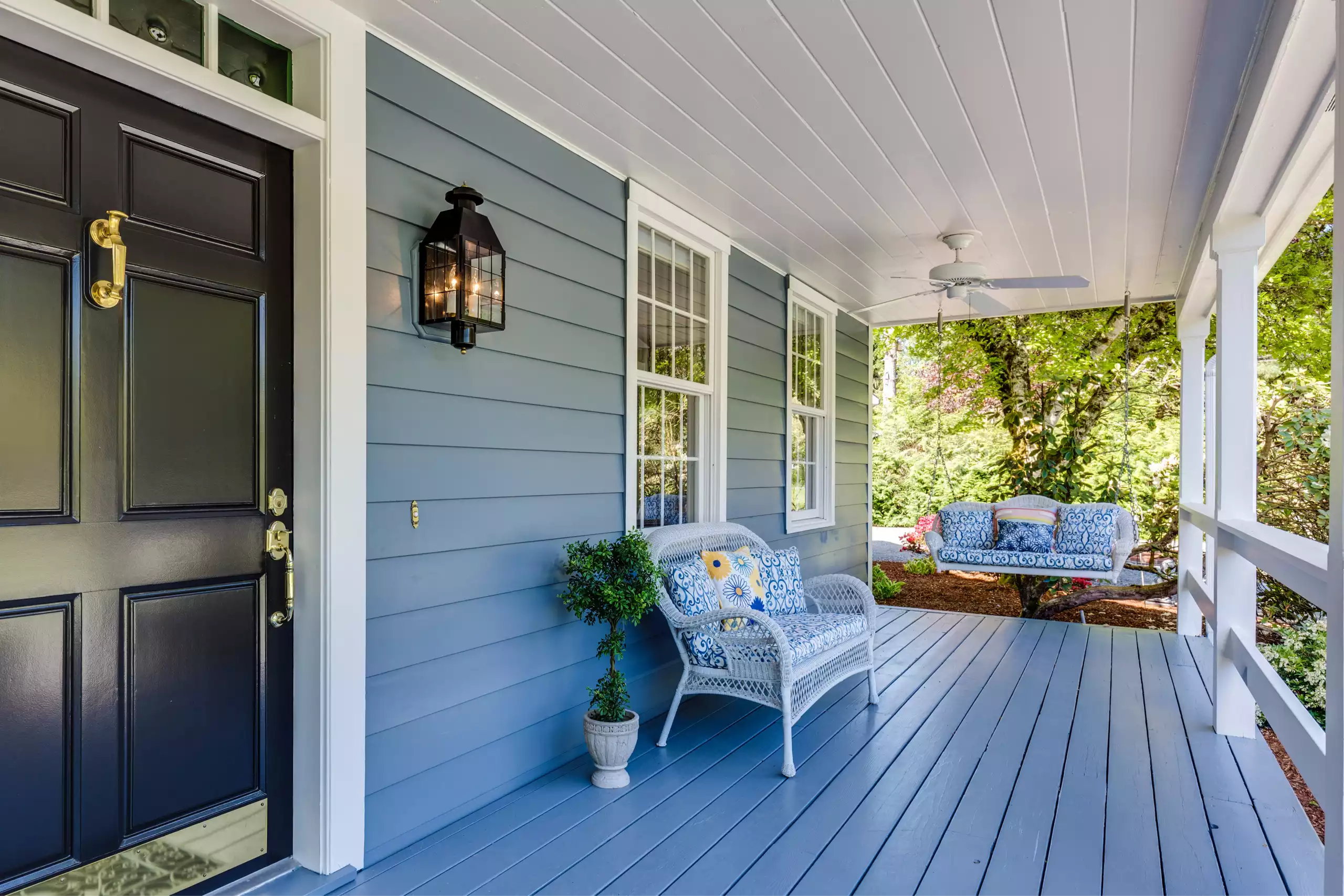Chat with the team

To calculate the square feet of a room, start by measuring the length and width of the room in feet using a tape measurer. Next, multiply the length by the width to calculate a room’s total square footage. So if a bedroom is 12 feet wide and 12 feet long, it is 144 square feet total (12 x 12 = 144 sqft).

Basically, the square footage of the room is the total area or size of the space. Often abbreviated as sqft or sq. ft, square feet is the most used unit of measurement for homes in the US.

In like fashion, square feet most accurately describe the size of rooms, walls, or an entire home. Moreover, sqft is commonly used in real estate and construction to budget supply and labor costs. According to the Census Bureau, the median size of a new single-family home was 2,356 square feet in 2021.

### Measuring Sqft in Odd-Shaped Rooms

Not every floor plan is a perfect square or rectangular room with 4 walls. Similarly, many rooms are odd-shaped and contain nooks or multiple angled walls.

Given that, the easy way to measure odd-shaped rooms is by separating areas into multiple squares or rectangles.

Using the floor plan above, you would first split the left portion of the space as a separate room in itself (section #1). Next, multiply the length by the width to get the total sqft (8 x 5 = 40 square feet).

Then measure the length and width of the larger, right-most room space (section #2). Again, multiply the width by the length of this room area to calculate its square footage (18 x 10 = 180 square feet).

Finally, add the sqft of section #1 and section #2 to calculate the total square footage of the oddly-shaped room (40 + 180 = 220 Sqft total).

### Most Common Square Footage Formulas:

• Length x Width = Total Square Footage Area Formula
• 1 Foot = 12 Inches
• 1 Square Foot = 144 Square Inches
• 3 Feet = 1 Yard
• 1 Square Yard (Metric) = 9 Square Foot (3 ft length x 3 ft width)
• 1 Meter = 3.28084 Feet
• 1 Square Meter = 10.7639 Sq. Feet

## Why Measure Square Footage?

Whether you’re a homeowner or contractor, knowing how to calculate the square feet in a room is vital to any home improvement project. No question, knowing the square footage of a house or room is helpful. Especially when deciding whether to DIY or hire a contractor based on the cost per sqft.

Not to mention, appraisers and realtors use the square footage of a residence to determine listing prices of homes and comparable properties. Moreover, square footage influences property taxes, mortgage appraisals, and home values. With this in mind, learning how to measure the square footage of your living area is crucial for homeowners.

So far, our experts have shown you how to calculate square feet of rooms. Now you’ll find out how to measure the total square footage of a house or wall fast.

Plus we share one trick to measure a home, wall, or room without a tape measurer or square footage calculator.

All in all, our goal is to make calculating the cost of house projects like painting, new flooring, or landscaping a breeze. Ultimately, saving you time and money as you measure sqft like a professional!

## Fastest Way To Measure Total Square Feet

If you don’t have a tape measurer handy, the easiest way to measure a room is simply to pace it out. Indeed, this is because each pace or wide step you take is about 3 feet.

Knowing this, you can get some idea of the length and width of a space by walking from one end of the room to the other.

First, start at one end of a room, heel to the baseboard, and count how many paces it takes to get to the opposite side. Since each pace is 3 feet, multiply the number of paces by three to calculate the total length of the room.

For example, a living room that takes 4 paces to walk from one wall to the opposite wall is approximately 12 feet long (4 x 3 = 12 ft).

Next, measure the wall perpendicular to where you started, heel to the baseboard, counting the paces to reach the opposite end. Again, multiply the number of paces by 3 to get the width of the area in feet (3 x 3 = 9 ft).

Finally, remember that the square footage of any room equals the length times the width of the room. As the last step, multiply 12 ft (length) by 9 ft (width) to get 108 square feet total in your living room.

## How To Calculate Square Footage of a Home

Now that we know how to come up with sqft per room, it’s time to learn how to calculate the total square footage of a home.

Whether you are measuring a single-family house, apartment, or condo, you’ll need the following supplies:

• Pencil and paper
• Calculator or phone
• Measuring tape or digital measuring tool

If your home is a perfect rectangle, simply measure the width and length in feet and multiply those two numbers. For example, if a one-story house is 30 feet wide by 60 feet long, then your property is 1,800 square feet (30 x 60 = 1,800).

However, most homes have more complicated floor plans.

In this scenario, follow these steps to get the total square footage of your house.

1. Draw a basic sketch of your home and label each room individually. Include hallways or foyers as separate “rooms” themselves.
2. Measure the length and width of each room in feet. Next, multiply length by width to calculate the room’s square footage. For instance: If a bedroom is 12 feet by 18 feet, its 216 square feet (12 x 18 = 216). Write the total square footage of each room in the corresponding spaces in your sketch.
3. Lastly, add all room measurements together to get the total square footage of your home. Don’t include spaces you couldn’t pace out or walk in. Also, exclude basement areas or unfinished spaces like attics.

Here is an example of what the final sketch will look like.

All in all, you don’t need to be an appraiser or real estate agent to know how to calculate the total sqft of a house.

Simply follow the steps above and you’ll be measuring your home’s square footage in no time. Just remember to measure each floor or level of your house separately. More often then not, the square footage of your 1st floor doesn’t match your 2nd floor. In this instance, measure each floor separately.

From family rooms to bathrooms, we found this awesome average room size cheat sheet to help you compare measurements.

## How To Calculate Square Feet of a Wall

Find the total square feet of a wall by multiplying ceiling height by wall length.

First, measure the total length of the wall across in feet using a tape measurer. Then measure the height of the wall from floor to ceiling. Finally, multiply the total wall length by its height to calculate the square footage of the wall.

For example, let’s say one wall measures 14 feet lengthwise from corner to corner with standard 8-foot ceilings. Next, multiply its length by ceiling height to get 112 square feet (14 x 8 = 122 sqft).

Do the same for each wall in a room and add them all up to calculate the total sqft of wall space in a room. Generally speaking, if all 4 walls in a room are equal lengths you can multiply the wall sqft by four.

### Square Footage Measurements of Windows or Doors

Optionally, you can subtract areas not covered like windows, doors, and closets from the total wall square footage. Without question, this method allows for more precise measurements. Indeed, this is useful when budgeting materials for interior painting, wallpaper, and drywall repairs.

No doubt, choosing the right interior design for your house is hard. Luckily, Improovy’s expert designers make it easy. Learn how to match wood flooring and wall paint colors in our latest post.

To save you time, here are the standard lengths and widths of windows, doors, and closets inside residential homes in the US. What’s more, we calculated the square footage of each for you already.

• Doors: 3 x 7 feet = 21 sqft
• Windows: 3 x 4 feet = 12 sqft
• Closets: 6 x 7 feet = 42 sqft

For example, say you have a 10-foot-long wall with lofted 12-foot ceilings in a dining room with 2 standard windows.

First, multiply the length and height of the wall to get the total square feet (10 x 12 = 120 sqft). Second, multiply the number of windows by the standard square feet of a window to get its square feet (2 windows x 12 = 24 sqft). Lastly, subtract the windows from the total wall square footage to get 96 square feet (120 – 24 = 96 sqft).

#### Length and Width Dimensions in Newer Homes

Many newer homes built after 2000 have 9 or 10-foot ceilings. Likewise, you’ll find lofted 13 to 18-foot ceilings in some larger modern houses.

In this case, we recommend using a laser measurer to safely measure a wall’s ceiling height. Particularly, ceiling height is an important factor in pricing for America’s most popular home improvement project, interior painting. However, for projects like installing hardwood flooring, ceiling height is less of a factor.

Often times taller ceilings also mean larger door, window, and closet sizes. To be sure, you can measure each window or door and subtract them individually.

Not to mention, tall ceilings multiply the complexity of painting a room. All in all, remember that bigger homes have larger room sizes and interior features.  No question, this will impact your calculations and housing measurements.

#### Measuring Ceiling Height Hack

The fastest way to find the ceiling height of a room is to measure the space between the top of a door to the ceiling. Given that the average door is 7 feet tall, an 8-foot ceiling would be 1-foot higher than the top of the door.

As an example, here are three photos comparing 8-foot ceilings versus 9 or 10-foot rooms.

Using this measuring hack, you can ballpark a room’s heights by simply looking at a photo. Certainly the fool-proof method is to measure ceiling height using a tape measurer or laser measuring tool. However, this tip can help save you time measuring multiple rooms.

## Square Feet Measurement FAQs

Here are a few of the most popular sq. ft calculation questions we get from homeowners and contractors.

Using generations of industry expertise and technology, Improovy allows homeowners to get their houses painted without the hassle.

We’ve hand-selected the best professional painters in the industry. You can trust that your interior painting or exterior crew is fully insured, licensed, and has the necessary skills to perform the job at the highest quality level.